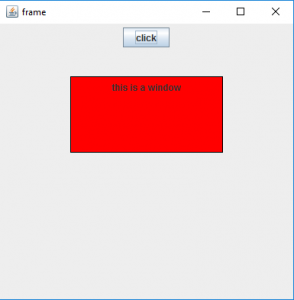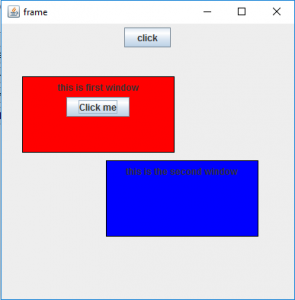# Java Swing | JWindow with examples

JWindow is a part of Java Swing and it can appear on any part of the users desktop. It is different from JFrame in the respect that JWindow does not have a title bar or window management buttons like minimize, maximize, and close, which JFrame has. JWindow can contain several components such as buttons and labels.
Constructor of the class are:

1. JWindow() : creates an empty Window without any specified owner
2. JWindow(Frame o) :creates an empty Window with a specified frame as its owner
3. JWindow(Frame o) : creates an empty Window with a specified frame as its owner
4. JWindow(Window o) : creates an empty Window with a specified window as its owner
5. JWindow(Window o, GraphicsConfiguration g) : creates an empty window with a specified window as its owner and specified graphics Configuration.
6. JWindow(GraphicsConfiguration g) :creates an empty window with a specified Graphics Configuration g.

Commonly used methods

1. setLayout(LayoutManager m) : sets the layout of the Window to specified layout manager
2. setContentPane(Container c) : sets the ContentPane property of the window
3. getContentPane() : get the container which is the ContentPane for this Window
5. isVisible(boolean b): sets the visibility of the Window, if value of the boolean is true then visible else invisible
6. update(Graphics g) : calls the paint(g) function
7. remove(Component c) : removes the component c
8. getGraphics() : returns the graphics context of the component.
9. getLayeredPane() : returns the layered pane for the window
10. setContentPane(Container c) :sets the content pane for the window
11. setLayeredPane(JLayeredPane l) : set the layered pane for the window
12. setRootPane(JRootPane r) : sets the rootPane for the window
13. setTransferHandler(TransferHandler n) : Sets the transferHandler property, which is a mechanism to support transfer of data into this component.
14. setRootPaneCheckingEnabled(boolean enabled) : Sets whether calls to add and setLayout are forwarded to the contentPane.
15. setRootPane(JRootPane root) :Sets the rootPane property of the window.
16. setGlassPane(Component glass) : Sets the glassPane property of the window.
17. repaint(long time, int x, int y, int width, int height): Repaints the specified rectangle of this component within time milliseconds.
18. remove(Component c): Removes the specified component from the window.
19. isRootPaneCheckingEnabled() : Returns whether calls to add and setLayout are forwarded to the contentPane or not .
20. getTransferHandler() : returns the transferHandler property.
21. getRootPane() : Returns the rootPane object for this window.
22. getGlassPane() : Returns the glassPane object for this window.
23. createRootPane() : Called by the constructor methods to create the default rootPane.
24. addImpl(Component co, Object c, int i) : Adds the specified child Component to the window.

The following programs will illustrate the use of JWindow

1. program to create a simple JWindow

 `// java Program to create a simple JWindow ` `import` `java.awt.event.*; ` `import` `java.awt.*; ` `import` `javax.swing.*; ` ` `  ` `  `class` `solveit ``extends` `JFrame ``implements` `ActionListener { ` ` `  `    ``// frame ` `    ``static` `JFrame f; ` ` `  `    ``// main class ` `    ``public` `static` `void` `main(String[] args) ` `    ``{ ` `        ``// create a new frame ` `        ``f = ``new` `JFrame(``"frame"``); ` ` `  `        ``// create a object ` `        ``solveit s = ``new` `solveit(); ` ` `  `        ``// create a panel ` `        ``JPanel p = ``new` `JPanel(); ` ` `  `        ``JButton b = ``new` `JButton(``"click"``); ` ` `  `        ``// add actionlistener to button ` `        ``b.addActionListener(s); ` ` `  `        ``// add button to panel ` `        ``p.add(b); ` ` `  `        ``f.add(p); ` ` `  `        ``// set the size of frame ` `        ``f.setSize(``400``, ``400``); ` ` `  `        ``f.show(); ` `    ``} ` ` `  `    ``// if button is pressed ` `    ``public` `void` `actionPerformed(ActionEvent e) ` `    ``{ ` `        ``String s = e.getActionCommand(); ` `        ``if` `(s.equals(``"click"``)) { ` `            ``// create a window ` `            ``JWindow w = ``new` `JWindow(f); ` ` `  `            ``// set panel ` `            ``JPanel p = ``new` `JPanel(); ` ` `  `            ``// create a label ` `            ``JLabel l = ``new` `JLabel(``"this is a window"``); ` ` `  `            ``// set border ` `            ``p.setBorder(BorderFactory.createLineBorder(Color.black)); ` ` `  `            ``p.add(l); ` `            ``w.add(p); ` ` `  `            ``// set background ` `            ``p.setBackground(Color.red); ` ` `  `            ``// setsize of window ` `            ``w.setSize(``200``, ``100``); ` ` `  `            ``// set visibility of window ` `            ``w.setVisible(``true``); ` ` `  `            ``// set location of window ` `            ``w.setLocation(``100``, ``100``); ` `        ``} ` `    ``} ` `} `

Output :1. program to create a multiple JWindow .( where one window is the owner of the other )

 `// java program to create a multiple  JWindow .( where one window is the owner of the other )< ` `import` `java.awt.event.*; ` `import` `java.awt.*; ` `import` `javax.swing.*; ` `class` `solveit ``extends` `JFrame ``implements` `ActionListener { ` ` `  `    ``// frame ` `    ``static` `JFrame f; ` ` `  `    ``// windows ` `    ``JWindow w, w1; ` ` `  `    ``// object of class ` `    ``static` `solveit s; ` ` `  `    ``// main class ` `    ``public` `static` `void` `main(String[] args) ` `    ``{ ` `        ``// create a new frame ` `        ``f = ``new` `JFrame(``"frame"``); ` ` `  `        ``// create a object ` `        ``s = ``new` `solveit(); ` ` `  `        ``// create a panel ` `        ``JPanel p = ``new` `JPanel(); ` ` `  `        ``JButton b = ``new` `JButton(``"click"``); ` ` `  `        ``// add actionlistener to button ` `        ``b.addActionListener(s); ` ` `  `        ``// add button to panel ` `        ``p.add(b); ` ` `  `        ``f.add(p); ` ` `  `        ``// set the size of frame ` `        ``f.setSize(``400``, ``400``); ` ` `  `        ``f.show(); ` `    ``} ` ` `  `    ``// if button is pressed ` `    ``public` `void` `actionPerformed(ActionEvent e) ` `    ``{ ` `        ``String s1 = e.getActionCommand(); ` `        ``if` `(s1.equals(``"click"``)) { ` `            ``// create a window ` `            ``w = ``new` `JWindow(f); ` ` `  `            ``// set panel ` `            ``JPanel p = ``new` `JPanel(); ` ` `  `            ``// create a label ` `            ``JLabel l = ``new` `JLabel(``"this is first window"``); ` ` `  `            ``// create a button ` `            ``JButton b = ``new` `JButton(``"Click me"``); ` ` `  `            ``// add Action listener ` `            ``b.addActionListener(s); ` ` `  `            ``// set border ` `            ``p.setBorder(BorderFactory.createLineBorder(Color.black)); ` ` `  `            ``p.add(l); ` `            ``p.add(b); ` `            ``w.add(p); ` ` `  `            ``// set background ` `            ``p.setBackground(Color.red); ` ` `  `            ``// setsize of window ` `            ``w.setSize(``200``, ``100``); ` ` `  `            ``// set visibility of window ` `            ``w.setVisible(``true``); ` ` `  `            ``// set location of window ` `            ``w.setLocation(``100``, ``100``); ` `        ``} ` `        ``else` `{ ` `            ``// create a window ` `            ``w1 = ``new` `JWindow(w); ` ` `  `            ``// set panel ` `            ``JPanel p = ``new` `JPanel(); ` ` `  `            ``// create a label ` `            ``JLabel l = ``new` `JLabel(``"this is the second window"``); ` ` `  `            ``// set border ` `            ``p.setBorder(BorderFactory.createLineBorder(Color.black)); ` ` `  `            ``p.add(l); ` ` `  `            ``w1.add(p); ` ` `  `            ``// set background ` `            ``p.setBackground(Color.blue); ` ` `  `            ``// setsize of window ` `            ``w1.setSize(``200``, ``100``); ` ` `  `            ``// set visibility of window ` `            ``w1.setVisible(``true``); ` ` `  `            ``// set location of window ` `            ``w1.setLocation(``210``, ``210``); ` `        ``} ` `    ``} ` `} `

Output :Note : the above programs might nor run in an online compiler please use an offline IDE

Attention reader! Don’t stop learning now. Get hold of all the important DSA concepts with the DSA Self Paced Course at a student-friendly price and become industry ready.

My Personal Notes arrow_drop_upCheck out this Author's contributed articles.

If you like GeeksforGeeks and would like to contribute, you can also write an article using contribute.geeksforgeeks.org or mail your article to contribute@geeksforgeeks.org. See your article appearing on the GeeksforGeeks main page and help other Geeks.

Please Improve this article if you find anything incorrect by clicking on the "Improve Article" button below.

Article Tags :
Practice Tags :

Be the First to upvote.

Please write to us at contribute@geeksforgeeks.org to report any issue with the above content.